10.8: Electronegativity and Polarity: Why Oil and Water Don’t Mix

Learning Objectives

• Explain how polar compounds differ from nonpolar compounds.
• Determine if a molecule is polar or nonpolar.
• Given a pair of compounds, predict which would have a higher melting or boiling point.

Bond Polarity

The ability of an atom in a molecule to attract shared electrons is called electronegativity. When two atoms combine, the difference between their electronegativities is an indication of the type of bond that will form. If the difference between the electronegativities of the two atoms is small, neither atom can take the shared electrons completely away from the other atom, and the bond will be covalent. If the difference between the electronegativities is large, the more electronegative atom will take the bonding electrons completely away from the other atom (electron transfer will occur), and the bond will be ionic. This is why metals (low electronegativities) bonded with nonmetals (high electronegativities) typically produce ionic compounds.

A bond may be so polar that an electron actually transfers from one atom to another, forming a true ionic bond. How do we judge the degree of polarity? Scientists have devised a scale called electronegativity, a scale for judging how much atoms of any element attract electrons. Electronegativity is a unitless number; the higher the number, the more an atom attracts electrons. A common scale for electronegativity is shown in Figure $$\PageIndex{1}$$.Figure $$\PageIndex{1}$$: Electronegativities of the Elements. Electronegativities are used to determine the polarity of covalent bonds.

The polarity of a covalent bond can be judged by determining the difference of the electronegativities of the two atoms involved in the covalent bond, as summarized in the following table:

Electronegativity Difference Bond Type
0–0.4 pure covalent
0.5–2.0 polar covalent
>2.0 likely ionic

Nonpolar Covalent Bonds

A bond in which the electronegativity difference is less than 1.9 is considered to be mostly covalent in character. However, at this point we need to distinguish between two general types of covalent bonds. A nonpolar covalent bond is a covalent bond in which the bonding electrons are shared equally between the two atoms. In a nonpolar covalent bond, the distribution of electrical charge is balanced between the two atoms.Figure $$\PageIndex{2}$$: A nonpolar covalent bond is one in which the distribution of electron density between the two atoms is equal.

The two chlorine atoms share the pair of electrons in the single covalent bond equally, and the electron density surrounding the $$\ce{Cl_2}$$ molecule is symmetrical. Also note that molecules in which the electronegativity difference is very small (<0.5) are also considered nonpolar covalent. An example would be a bond between chlorine and bromine ($$\Delta$$EN $$=3.0 - 2.8 = 0.2$$).

Polar Covalent Bonds

A bond in which the electronegativity difference between the atoms is between 0.5 and 2.0 is called a polar covalent bond. A polar covalent bond is a covalent bond in which the atoms have an unequal attraction for electrons and so the sharing is unequal. In a polar covalent bond, sometimes simply called a polar bond, the distribution of electrons around the molecule is no longer symmetrical.Figure $$\PageIndex{3}$$: In the polar covalent bond of $$\ce{HF}$$, the electron density is unevenly distributed. There is a higher density (red) near the fluorine atom, and a lower density (blue) near the hydrogen atom.

An easy way to illustrate the uneven electron distribution in a polar covalent bond is to use the Greek letter delta $$\left( \delta \right)$$.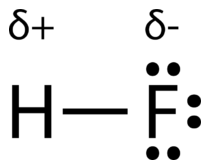Figure $$\PageIndex{4}$$: Use of $$\delta$$ to indicate partial charge.

The atom with the greater electronegativity acquires a partial negative charge, while the atom with the lesser electronegativity acquires a partial positive charge. The delta symbol is used to indicate that the quantity of charge is less than one. A crossed arrow can also be used to indicate the direction of greater electron density.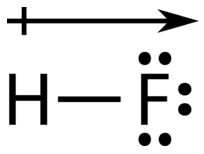Figure $$\PageIndex{5}$$: Use of crossed arrow to indicate polarity.

Electronegativity differences in bonding using Pauling scale. Differences in electronegativity classify bonds as covalent, polar covalent, or ionic.

Example $$\PageIndex{1}$$: Bond Polarity

What is the polarity of each bond?

1. C–H
2. O–H

Solution

Using Figure $$\PageIndex{1}$$, we can calculate the difference of the electronegativities of the atoms involved in the bond.

1. For the C–H bond, the difference in the electronegativities is 2.5 − 2.1 = 0.4. Thus we predict that this bond will be nonpolar covalent.
2. For the O–H bond, the difference in electronegativities is 3.5 − 2.1 = 1.4, so we predict that this bond will be polar covalent.

Exercise $$\PageIndex{1}$$

What is the polarity of each bond?

1. Rb–F
2. P–Cl

likely ionic

polar covalent

Molecular Polarity

To determine if a molecule is polar or nonpolar, it is generally useful to look at Lewis structures. Nonpolar compounds will be symmetric, meaning all of the sides around the central atom are identical—bonded to the same element with no unshared pairs of electrons. Polar molecules are asymmetric, either containing lone pairs of electrons on a central atom or having atoms with different electronegativities bonded. This works pretty well, as long as you can visualize the molecular geometry. That's the hard part. To know how the bonds are oriented in space, you have to have a strong grasp of Lewis structures and VSEPR theory. Assuming that you do, you can look at the structure of each one and decide if it is polar or not, whether or not you know the individual atom's electronegativity. This is because you know that all bonds between dissimilar elements are polar, and in these particular examples, it doesn't matter which direction the dipole moment vectors are pointing (out or in).

A polar molecule is a molecule in which one end of the molecule is slightly positive, while the other end is slightly negative. A diatomic molecule that consists of a polar covalent bond, such as $$\ce{HF}$$, is a polar molecule. The two electrically charged regions on either end of the molecule are called poles, similar to a magnet having a north and a south pole. A molecule with two poles is called a dipole (see figure below). Hydrogen fluoride is a dipole.Figure $$\PageIndex{6}$$: A dipole is any molecule with a positive end and a negative end, resulting from unequal distribution of electron density throughout the molecule.

For molecules with more than two atoms, the molecular geometry must also be taken into account when determining if the molecule is polar or nonpolar. The figure below shows a comparison between carbon dioxide and water. Carbon dioxide $$\left( \ce{CO_2} \right)$$ is a linear molecule. The oxygen atoms are more electronegative than the carbon atom, so there are two individual dipoles pointing outward from the $$\ce{C}$$ atom to each $$\ce{O}$$ atom. However, since the dipoles are of equal strength and are oriented this way, they cancel out and the overall molecular polarity of $$\ce{CO_2}$$ is zero.

Water is a bent molecule because of the two lone pairs on the central oxygen atom. The individual dipoles point from the $$\ce{H}$$ atoms toward the $$\ce{O}$$ atom. Because of the shape, the dipoles do not cancel each other out and the water molecule is polar. In the figure below, the net dipole is shown in blue and points upward.Figure $$\PageIndex{7}$$: The molecular geometry of a molecule affects its polarity. Each CO bond has a dipole moment, but they point in opposite directions so that the net CO2 molecule is nonpolar. In contrast, water is polar because the OH bond moments do not cancel out.

Some other molecules are shown in the figure below. Notice that a tetrahedral molecule such as $$\ce{CH_4}$$ is nonpolar. However, if one of the peripheral $$\ce{H}$$ atoms is replaced with another atom that has a different electronegativity, the molecule becomes polar. A trigonal planar molecule $$\left( \ce{BF_3} \right)$$ may be nonpolar if all three peripheral atoms are the same, but a trigonal pyramidal molecule $$\left( \ce{NH_3} \right)$$ is polar.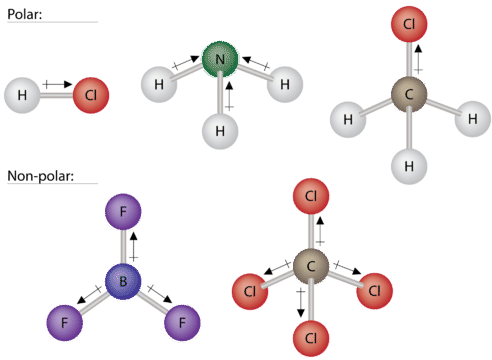Figure $$\PageIndex{8}$$: Some examples of polar and nonpolar molecules based on molecular geometry.

To summarize, to be polar, a molecule must:

1. Contain at least one polar covalent bond.
2. Have a molecular structure such that the sum of the vectors of each bond dipole moment do not cancel.

Steps to Identify Polar Molecules

1. Draw the Lewis structure.
2. Figure out the geometry (using VSEPR theory).
3. Visualize or draw the geometry.
4. Find the net dipole moment (you don't have to actually do calculations if you can visualize it).
5. If the net dipole moment is zero, it is non-polar. Otherwise, it is polar.

Properties of Polar Molecules

Polar molecules tend to align when placed in an electric field with the positive end of the molecule oriented toward the negative plate and the negative end toward the positive plate (Figure $$\PageIndex{14}$$). We can use an electrically charged object to attract polar molecules, but nonpolar molecules are not attracted. Also, polar solvents are better at dissolving polar substances, and nonpolar solvents are better at dissolving nonpolar substances.Figure $$\PageIndex{9}$$: (a) Molecules are always randomly distributed in the liquid state in the absence of an electric field. (b) When an electric field is applied, polar molecules like HF will align to the dipoles with the field direction. (OpenStax CC-BY-SA);

While molecules can be described as "polar covalent" or "ionic", it must be noted that this is often a relative term, with one molecule simply being more polar or less polar than another. However, the following properties are typical of such molecules. Polar molecules tend to:

• have higher melting points than nonpolar molecules
• have higher boiling points than nonpolar molecules
• be more soluble in water (dissolve better) than nonpolar molecules
• have lower vapor pressures than nonpolar molecules

Example $$\PageIndex{2}$$:

Label each of the following as polar or nonpolar.

1. Water, H2O:2. Methanol, CH3OH: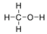3. Hydrogen Cyanide, HCN:4. Oxygen, O2:5. Propane, C3H8: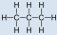Solution

1. Water is polar. Any molecule with lone pairs of electrons around the central atom is polar.
2. Methanol is polar. This is not a symmetric molecule. The $$\ce{-OH}$$ side is different from the other 3 $$\ce{-H}$$ sides.
3. Hydrogen cyanide is polar. The molecule is not symmetric. The nitrogen and hydrogen have different electronegativities, creating an uneven pull on the electrons.
4. Oxygen is nonpolar. The molecule is symmetric. The two oxygen atoms pull on the electrons by exactly the same amount.
5. Propane is nonpolar, because it is symmetric, with $$\ce{H}$$ atoms bonded to every side around the central atoms and no unshared pairs of electrons.

Exercise $$\PageIndex{2}$$

Label each of the following as polar or nonpolar.

a. SO3

b. NH3

nonpolar